## Python 机器学习实战 —— 监督学习（上）

1.1 机器学习简介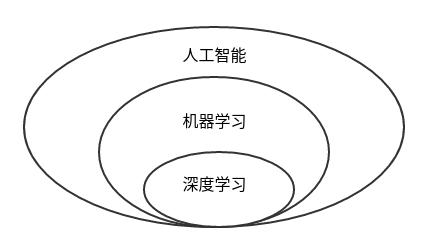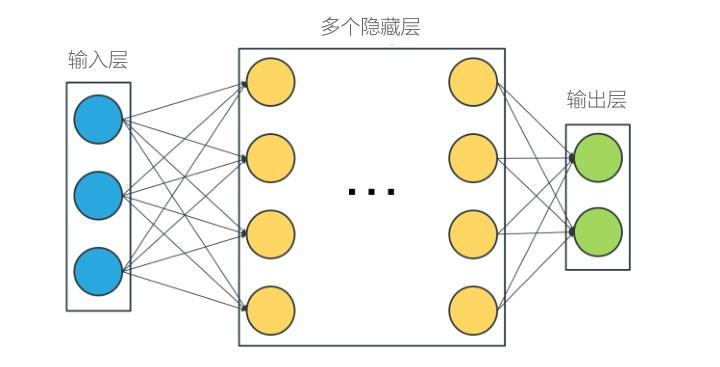1.2  机器学习分类

1.2.1  监督学习

1.2..2  无监督学习

二、基本概念

2.1 分类与回归的区别

2.2 数据划分

2.3  过拟合与欠拟合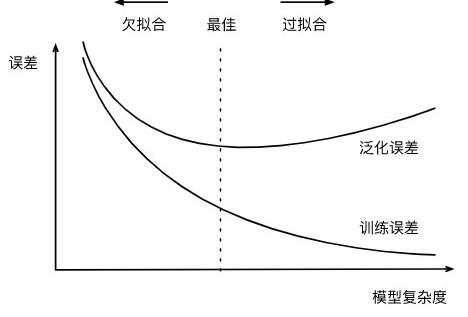2.4 损失函数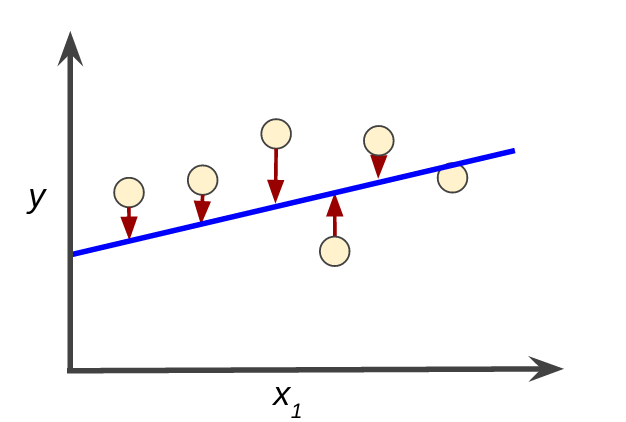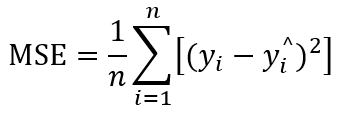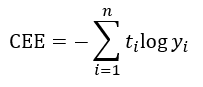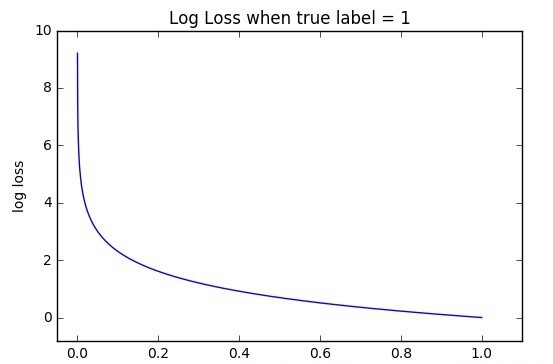2.4.1 最小二乘法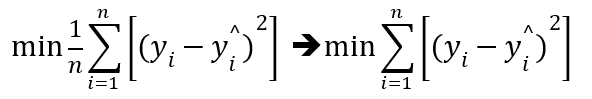代入  y=a*x+b  后，计算公式可得到转换成下图，由于计算的是平方和，所以表达式必然存在最小值，通过偏导数为 0 时得极值的原理，可得到计算公式：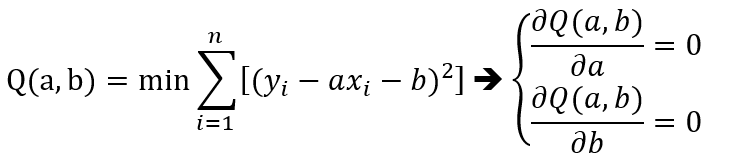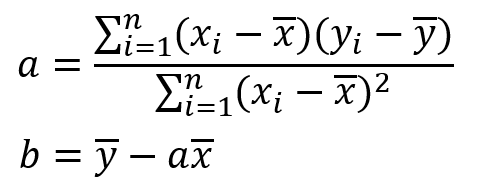2.4.2 梯度下降法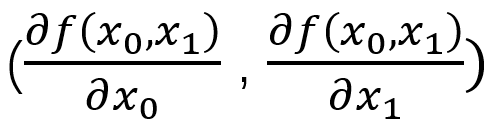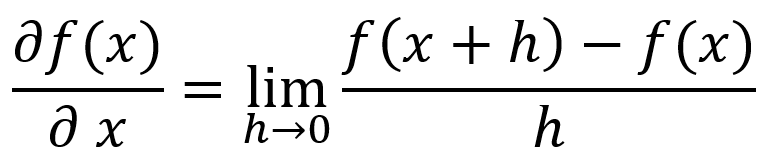1 class gradinet_drop():
2     def __init__(self):
3         return
4
5     def f(self, x):
6         return x*x+1
7
8     def polt_line(self):
9         # 画出 y=x*x+1 图
10         x = np.linspace(-5, 5, 100)
11         plt.plot(x.reshape(-1, 1), self.f(x))
12         plt.xlabel('x')
13         plt.ylabel('f(x)')
14
15     def tangent(seft,x,h=0.0001):
16         #根据 y=ax+b 直线公式
17         #求导数，即切线斜率
18         a=(seft.f(x+h)-seft.f(x))/h
19         #求截距
20         b=seft.f(x+h)-a*x+h
21         #划出切线
22         seft.polt_tangent(a,b)
23         return a
24
25     def polt_tangent(self,a,b):
26         #划出直线 y=ax+b
27         x = np.linspace(-5, -1, 100)
28         y=a*x+b
29         plt.plot(x.reshape(-1,1),y,'--')
30
33 # 分别以 h 等于 -0.3，-0.2，-0.001 ,0.0001求斜率
34 # 将发现 h 越小，所得斜率越接于近切线斜率
35 for h in [-0.3,-0.2,0.001,0.0001]:
37 plt.show()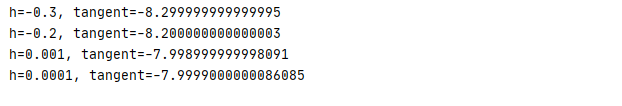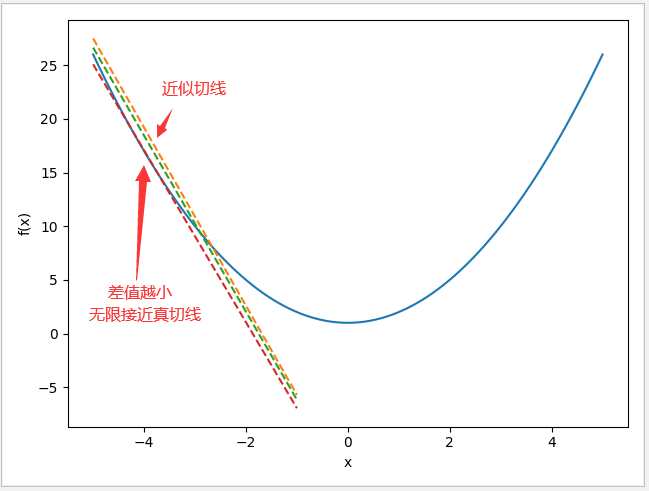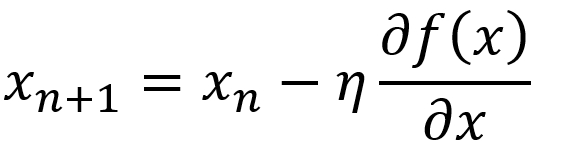1 class gradinet_drop():
2     def __init__(self):
3         return
4
5     def f(self, x):
6         return x*x+1
7
8     def polt_line(self):
9         # 画出 y=x*x+1 图
10         x = np.linspace(-5, 5, 100)
11         plt.plot(x.reshape(-1, 1), self.f(x))
12         plt.xlabel('x')
13         plt.ylabel('f(x)')
14
15     def tangent(seft,x,h=0.0001):
16         #根据 y=ax+b 直线公式
17         #求导数（切线斜率）
18         a=(seft.f(x+h)-seft.f(x))/h
19         #求截距
20         b=seft.f(x+h)-a*x+h
21         #随机画出切线
22         n=np.random.randint(100)
23         if(n==5):
24             seft.polt_tangent(a,b)
25         return a
26
27     def polt_tangent(self,a,b):
28         #划出切线 y=ax+b
29         x = np.linspace(-5, 1, 100)
30         y=a*x+b
31         plt.plot(x.reshape(-1,1),y,'--')
32
34         #学习率默认为0.01，默认重复1000次
35         for i in range(n):
36             x-=seft.tangent(x)*rate
37             print('x={0},f(x)={1},tangent slope={2}'.format(x,seft.f(x),seft.tangent(x)))
38
41 #使用学习率默认0.01，默认重复1000次求出鞍点
43 plt.show()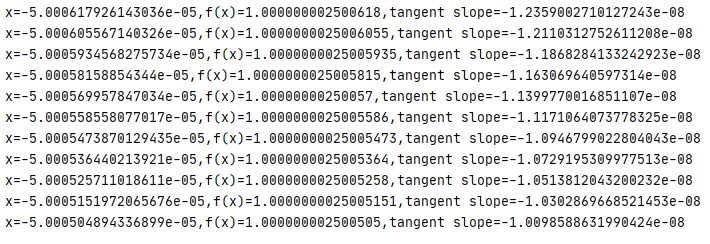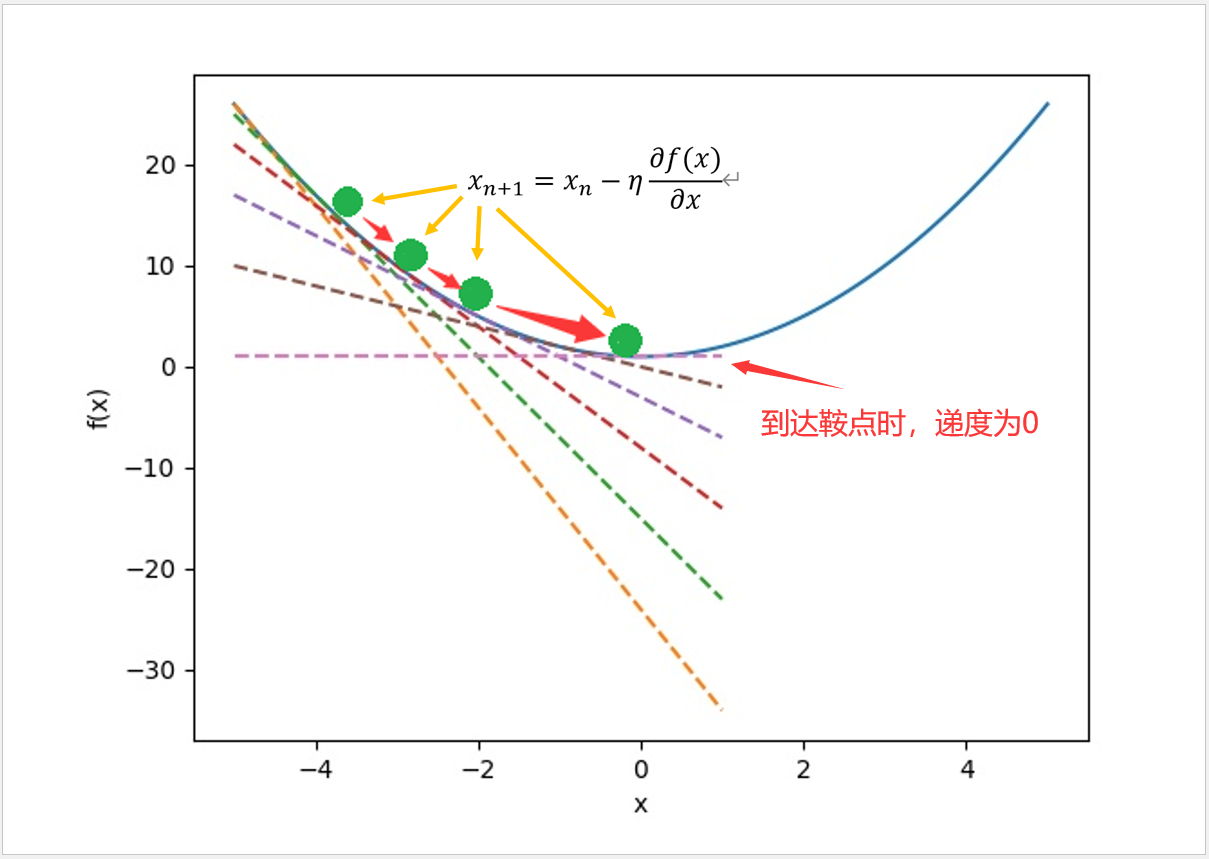1 class gradinet_drop():
2     def __init__(self,train_x,train_y):
3         #定义测试数据
4         self.x=train_x
5         self.y=train_y
6         return
7
8     def mes(self,a,b):
9         #均方误差计算公式
10         return mean((self.y-a*self.x-b)**2)
11
12     def partial_derivative(seft):
13         #求 mes(a,b)对 a,b 的偏导数
14         partial_derivative_a=sp.diff(seft.mes(a,b), a)
15         partial_derivative_b=sp.diff(seft.mes(a,b),b)
16         return [partial_derivative_a,partial_derivative_b]
17
19         #学习率默认为0.01，默认重复1000次
20         #把 y=a*x+b 参数 a,b 的初始值设为 1，1
21         a1=1
22         b1=1
23         #默认训练1000次，找到最小均方误差时的 a,b 值
24         for i in range(n):
25             deri=seft.partial_derivative()
26             a1-=deri.subs({a:a1,b:b1})*rate
27             b1-=deri.subs({a:a1,b:b1})*rate
28         #输出直线参数 a,b 值
29         print('y=a*x=b\n  a={0},b={1}'.format(a1,b1))
30         return [a1,b1]
31
32     def polt_line(self,param):
33         #根据a,b参数值划出直线 y=a*x+b
34         x = np.linspace(-3, 3, 100)
35         y=param*x+param
36         plt.plot(x,y,20)
37         plt.legend(['train data','line'])
38
39 #输入测试数据
40 X,y=dataset.make_regression(n_features=1,noise=5)
42 #画出数据点
43 plt.plot(X,y,'.')
44 #训练数据找出最小均方误差时的参数 a,b 值
46 #画出训练后的直线
48 plt.show()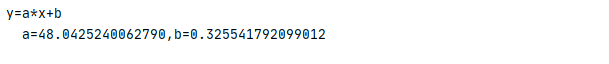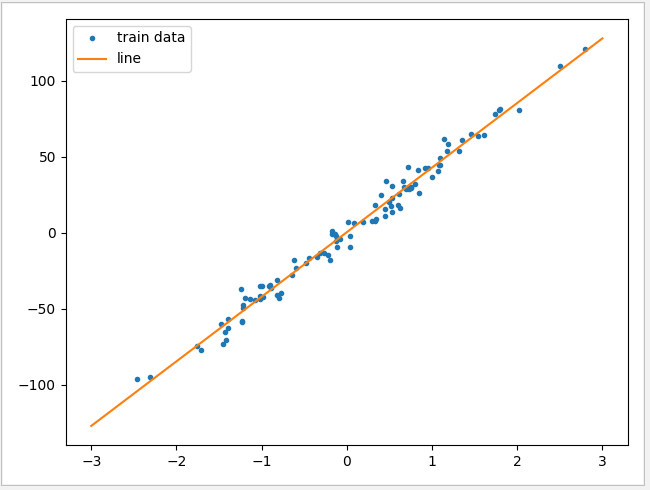Scikit-Learn 是目录最常用的机器学习库，所以在本文当中大部实例都是运用当中的方法完成。

3.1  train_test_split 方法

train_test_split( * arrays,  test_size=None,   train_size=None, random_state=None, shuffle=True,stratify=None)

• *arrays：可以是列表、numpy数组、scipy稀疏矩阵或pandas的数据框
• test_size：可以为浮点、整数或None，默认为None

①若为浮点时，表示测试集占总样本的百分比

②若为整数时，表示测试样本样本数

③若为None时，test size自动设置成0.25

• train_size：可以为浮点、整数或None，默认为None

①若为浮点时，表示训练集占总样本的百分比

②若为整数时，表示训练样本的样本数

③若为None时，train_size自动被设置成0.75

• random_state：可以为整数、RandomState实例或None，默认为None

①若为None时，每次生成的数据都是随机，可能不一样

②若为整数时，每次生成的数据都相同

• stratify可以为类似数组或None

①若为None时，划分出来的测试集或训练集中，其类标签的比例也是随机的

②若不为None时，划分出来的测试集或训练集中，其类标签的比例同输入的数组中类标签的比例相同，用于处理不均衡数据集

1     X,y=make_wave(1000)
2     X_train,X_test,y_train,y_test=train_test_split(X,y)

train_size 与 test_size 默认值为75% 与 25% ，所以上面的例子与下面例子得出结果相同

1     X,y=make_wave(1000)
2     X_train,X_test,y_train,y_test=train_test_split(X,y,train_size=0.75,test_size=0.25)

1     X,y=make_wave(1000)
2     X_train,X_test,y_train,y_test=train_test_split(X,y,random_state=0)

3.2 predict 方法与 accuracy_score 方法

accuracy_score 主要用于对比预测结果的准备率

 1     X,y=make_blobs(n_samples=150,n_features=2)
2     #划分训练数据与测试数据
3     X_train,X_test,y_train,y_test=train_test_split(X,y)
4     #绑定模型
5     knn_classifier=KNeighborsClassifier(n_neighbors=7)
6     #输入训练数据
7     knn_classifier.fit(X_train,y_train)
8     #运行测试数据
9     y_model=knn_classifier.predict(X_test)
10     #把运行结果与测试结果进行对比
11     print(accuracy_score(y_test,y_model))        

3.3 score 方法

R^2=1，则表示模型与数据完成吻合，如果R^2为负值，侧表示模型性能非常差。

1     X,y=make_wave(1000)
2     X_train,X_test,y_train,y_test=train_test_split(X,y,random_state=0)
3     linearReg=LinearRegression()
4     linearReg.fit(X_train,y_train)
5     print(linearReg.score(X_train,y_train))

3.4 numpy.meshgrid 方法

1 x=np.array([1,2,3])
2 y=np.array([4,5])
3 #显示网格矩阵坐标
4 X,Y=np.meshgrid(x,y)
5 print('X:\n '+str(X))
6 print('Y:\n '+str(Y))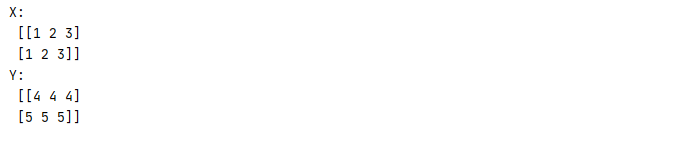3.5 contour 和 contourf 方法

pyplot 的 contour 和 contourf 方法都是画三维等高线图的，不同点在于contour 是绘制轮廓线，contourf 会填充轮廓。本文在分类模型中，将会大量地使用此方法，让各位可以更直观的看到模型预测值的分布。
pyplot.contour (X，Y，Z，levels , linestyles，alpha，cmap )

X、Y :  必须是 2-D，且形状与 Z 相同。
Z：绘制轮廓的高度值。
levels：int 数组，可选填，

linestyles：数组，可选填，确定线条形状，例如：linestyles = [ '--' , '-' , '=' ] 。
alpha: 透明度
cmap：颜色

1 def f(x,y):
2     return 3*x**2+x+y**+1
3
4 x=np.linspace(-3,3,100)
5 y=np.linspace(-3,15,100)
6 X,Y=np.meshgrid(x,y)
7 plt.contourf(X,Y,f(X,Y))
8 plt.show()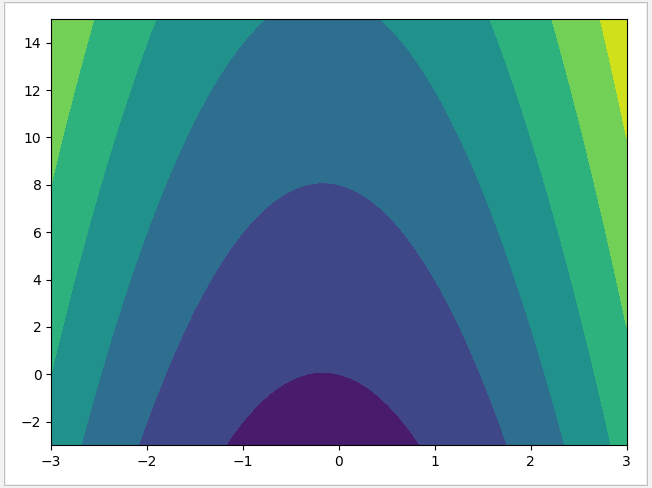3.6 decision_function 方法

 1     # 训练数据
2     X,y=dataset.make_blobs(centers=2,random_state=2,n_features=2)
3     # 使用 LinearSVC 模型，设置 C=100
4     linear=LinearSVC(C=100)
5     linear.fit(X,y)
6     # 画出数据点
7     plt.scatter(X[:,0],X[:,1],c=y,marker='^',s=50)
8     # 建立网格数据
9     xx=np.linspace(-5,6,2)
10     yy=np.linspace(-13,3,2)
11     XX,YY=np.meshgrid(xx,yy)
12     ZZ=np.c_[XX.ravel(),YY.ravel()]
13     # 根据网络数据推算出预测值
14     zz=linear.decision_function(ZZ)
15     aa=linear.predict(ZZ)
16     print('predict: '+str(aa))
17     print('decision_function:'+str(zz))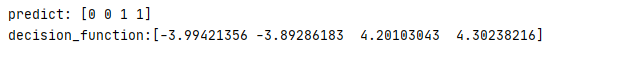predict 得到的最终的分类结果：0或1，而 decision_function 得到的是与分隔平面的距离，正值代表分类是 1，负值代表分类为 0，值越大代表与分隔面的距离越大。

3.7 confusion_matrix 混淆矩阵

 1     (X_train, y_train), (X_test, y_test) = keras.datasets.mnist.load_data()
2     # 把28*28图像数据进行转换
3     X_train = X_train.reshape(-1, 784)
4     X_test = X_test.reshape(-1, 784)
5     # 使用SGDClassfier模式，使用多核计算,学习率为0.01
6     sgd_classifier = SGDClassifier(learning_rate='constant', early_stopping=True,
7                                    eta0=0.001, loss='squared_hinge',
8                                    n_jobs=-1, max_iter=10000)
9     sgd_classifier.fit(X_train, y_train)
10     y_model=sgd_classifier.predict(X_test)
11     #建立混淆矩阵
12     mat=confusion_matrix(y_test,y_model)
13     #显示分类数据的测试分布
14     heatmap(mat,square=True,annot=True,fmt='d')
15     plt.show()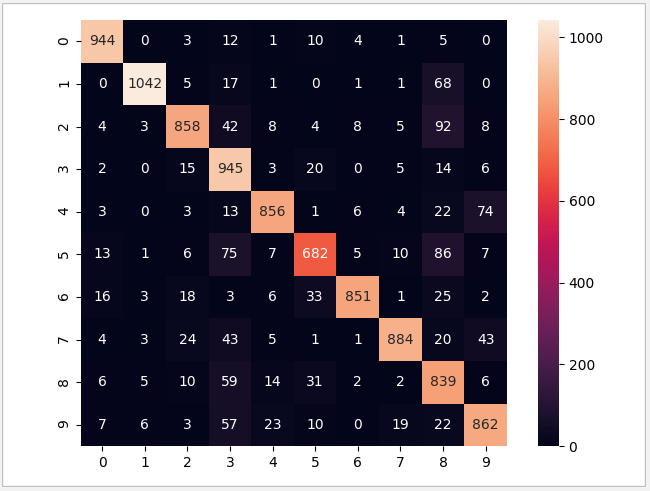3.8 validation_curve 验证曲线

validation_curve(estimator, X, y, *, param_name, param_range, groups=None,
cv=None, scoring=None, n_jobs=None, pre_dispatch="all",
verbose=0, error_score=np.nan, fit_params=None)

• estimator：实现了fit 和 predict 方法的对象
• X : 训练的向量
• y : 目标相对于X分类或回归
• param_name:将被改变的变量名称
• param_range:param_name对应的变量的取值
• cv:如果传入整数，测试数据将分成对应的分数，其中一份作为cv集，其余n-1作为traning（默认为3份）

 1     # validation_curve 绑定 SVC
3     param_range = np.logspace(-6, -1, 5)
4     train_scores, test_scores = validation_curve(
5         SVC(), X, y, param_name="gamma", param_range=param_range,
6         scoring="accuracy", n_jobs=1)
7     #训练数据与测试数据的平均得分
8     train_scores_mean = np.mean(train_scores, axis=1)
9     test_scores_mean = np.mean(test_scores, axis=1)
10     #显示数据
11     plt.title("Validation Curve with SVM")
12     plt.xlabel(r"$\gamma$")
13     plt.ylabel("Score")
14     plt.ylim(0.0, 1.1)
15     lw = 2
16     plt.semilogx(param_range, train_scores_mean, label="Training score",
17                  color="darkorange", lw=lw)
18     plt.semilogx(param_range, test_scores_mean, label="Cross-validation score",
19                  color="navy", lw=lw)
20     plt.legend(loc="best")
21     plt.show()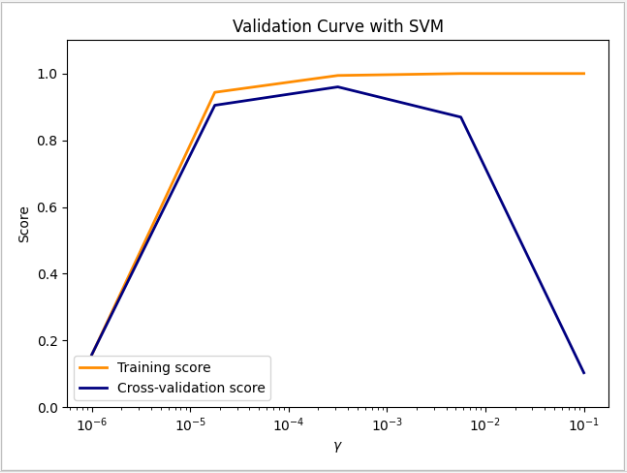w 为斜率，也被称为权重被保存在 coef_ 属性当中;
k 为载矩也称偏移量，被保存于 intercept_ 属性当中。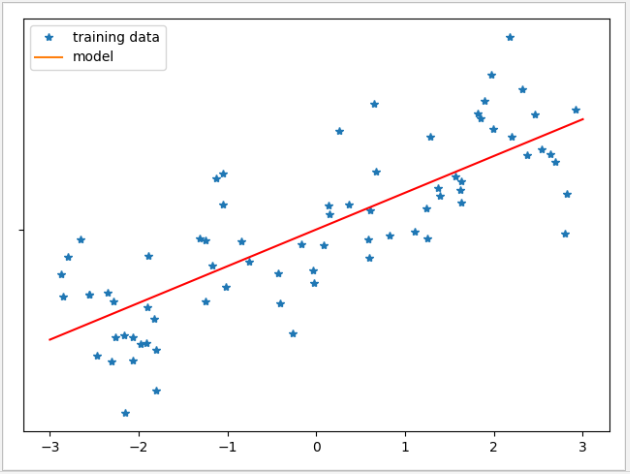y = w * x + w * x + w * x + w * x + ...... + w[n] * x[n] + k

4.1 线性回归 LinearRegression

LinearRegression 是最简单的的线性模型，此模型就是通过第二节介绍的最小二乘法，找出参数 w 与 k，使得训练集与预测集的均方误差 MSE 最小，最终确定 w 与 k 值。

 1 def linear_regression_test():
2     #测试数据
3     line=np.linspace(-3,3,50)
4     datasets.make_regression(n_features=1,noise=35，random_state=1)
5     X_train,X_test,y_train,y_test=train_test_split(X,y)
6     #线性回归模型训练
7     linear=LinearRegression()
8     linear.fit(X_train,y_train)
9     #准确率
10     print('train data prec:'+str(linear.score(X_train,y_train)))
11     print('test data prec:'+str(linear.score(X_test,y_test)))
12     #斜率与截距
13     print('coef:'+str(linear.coef_))
14     print('intercept:'+str(linear.intercept_))
15     #图形显示
16     plt.plot(X_train,y_train,'*','')
17     result=linear.predict(line.reshape(-1,1))
18     plt.plot(line,result,'r')
19     plt.legend(['training data','model'])
20     plt.show()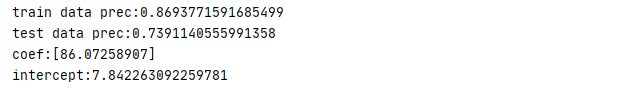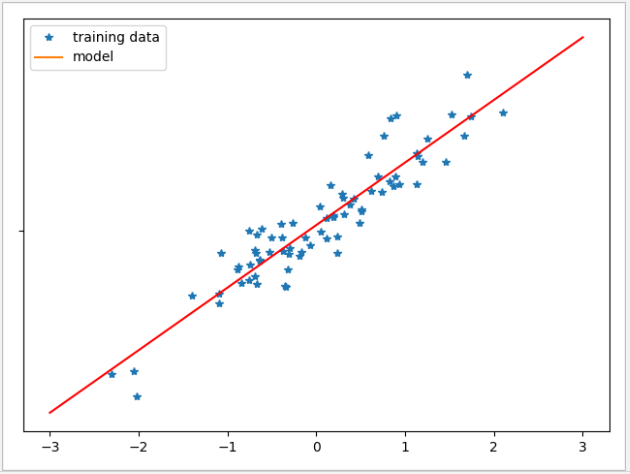LinearRegression 模型比较简单，不需要任何参数，但因此也无法调节模型的复杂程度，可以看到训练数据与测试数据的准确都不高。在实际应用中，LinearRegression 模型的应用场景并不多。

4.2 Ridge 岭回归

Ridge 构造函数

1 class Ridge(MultiOutputMixin, RegressorMixin, _BaseRidge):
2     @_deprecate_positional_args
3     def __init__(self, alpha=1.0, *, fit_intercept=True, normalize=False,
4                  copy_X=True, max_iter=None, tol=1e-3, solver="auto",
5                  random_state=None):
6     ......

• alpha 是正则项系数，初始值为1，数值越大，则对复杂模型的惩罚力度越大。
• fit_intercept：bool类型，默认为True，表示是否计算截距 ( 即 y=wx+k 中的 k )。
• normalize：bool类型，默认为False，表示是否对各个特征进行标准化(默认方法是：减去均值并除以L2范数)，推荐设置为True。如果设置为False，则建议在输入模型之前，手动进行标准化。当fit_intercept设置为False时，将忽略此参数。
• copy_X：默认值为True，代表 x 将被复制，为 False 时，则 x 有可能被覆盖。
• max_iter：默认值为None，部分求解器需要通过迭代实现，这个参数指定了模型优化的最大迭代次数。
• tol：默认为小数点后3位，代表求解方法精度
• solver：求解优化问题的算法，默认值 auto，可以根据数据类型选择最合适的算法。可选的算法有：

1).svd:采用奇异值分解的方法来计算。

2).cholesky:采用scipy.linalg.solve函数求得闭式解。

3).sparse_cg:采用scipy.sparse.linalg.cg函数来求取最优解。

4).lsqr:使用scipy.sparse.linalg.lsqr求解，它是最快的。

5).sag:使用随机平均梯度下降，当n_samples和n_features都较大时，通常比其他求解器更快。

• random_state：随机数种子，推荐设置一个任意整数，同一个随机值，模型可以复现。

Ridge 使用了 L2 正则化规范，对模型系数 w 进行约束，使每个特征的 w 尽可能的小，避免过度拟合。 相比 LinearRegression ，可见 Ridge 使用 L2 正则化规范后，分数有进一步的提升。

 1 def ridge_test():
2     #测试数据
3     line=np.linspace(-3,3,100)
4     X,y=datasets.make_regression(n_features=1,noise=35,random_state=1)
5     X_train,X_test,y_train,y_test=train_test_split(X,y)
6     #岭回归模型
7     ridge=Ridge(alpha=1)
8     ridge.fit(X_train,y_train)
9     #计算准确率
10     print('train data prec:'+str(ridge.score(X_train,y_train)))
11     print('test data prec:'+str(ridge.score(X_test,y_test)))
12     #斜率与截距
13     print('coef:'+str(ridge.coef_))
14     print('intercept:'+str(ridge.intercept_))
15     # 图形显示
16     plt.plot(X_train, y_train, '*', '')
17     plt.legend(['training data', 'model'])
18     result = ridge.predict(line.reshape(-1, 1))
19     plt.plot(line, result, 'r')
20     plt.show()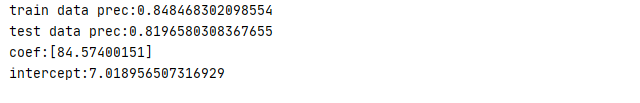Ridge 模型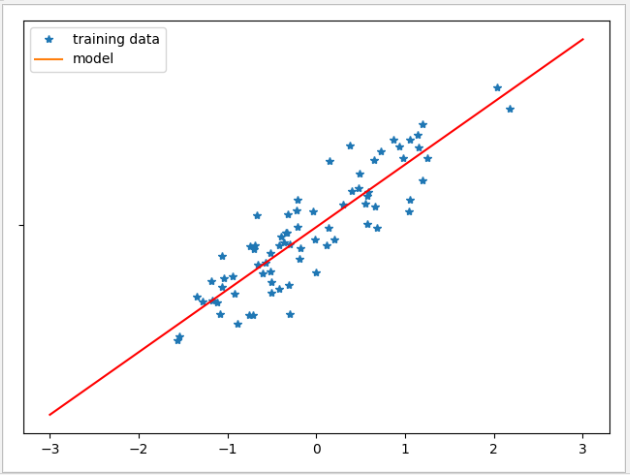alpha 越大，w 会更趋向于零，因此泛化性能越高，但训练集上的性能会降低，在数据量较大的时候需要权衡利弊。
alpha 越小，w 的限制就会越小，训练集上的性能就会越高。要注意的是如果把 alpha 调到接近于 0，那 L2 正则化基本不起作用，Ridge 的计算结果将接近于 LinearRegression。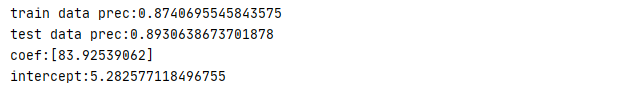4.3 Lasso 模型

Lasso 跟 Ridge 一样都是线性回归模型，两者的主要区别在 Ridge 默认是使用 L2 正则化，而 Lasso 使用的是 L1 正则化规范。L1 会把参数控制趋向于0，从而抽取更有关键代表性的几个特征。
Lasso的构造函数

 1 class Lasso(ElasticNet):
2     @_deprecate_positional_args
3     def __init__(self, alpha=1.0, *, fit_intercept=True, normalize=False,
4                  precompute=False, copy_X=True, max_iter=1000,
5                  tol=1e-4, warm_start=False, positive=False,
6                  random_state=None, selection='cyclic'):       ......

• alpha 是正则项系数，初始值为1，数值越大，则对复杂模型的惩罚力度越大。
• fit_intercept：bool类型，默认为True，表示是否计算截距(即y=wx+k中的k)。
• normalize：bool类型，默认为False，表示是否对各个特征进行标准化(默认方法是：减去均值并除以L2范数)，推荐设置为True。如果设置为False，则建议在输入模型之前，手动进行标准化。当fit_intercept设置为False时，将忽略此参数。
• precompute：默认值为 Falise，表示是否使用预计算的 Gram 矩阵来加速计算。如果设置为 auto 代表让计算机来决定。Gram 矩阵也可以作为参数传递。对于稀疏输入这个选项总是正确的，用于保持稀疏性。
• copy_X：默认值为True，代表 x 将被复制，为 False 时，则 x 有可能被覆盖。
• max_iter：默认值为10000，部分求解器需要通过迭代实现，这个参数指定了模型优化的最大迭代次数。
• tol：默认为小数点后 4 位，代表求解方法精度。
• warm_start：默认值为 False，当设置为True时，重用之前调用的解决方案作为初始化，否则，只需要删除前面的解决方案。
• positive：默认值为 False，当设置为 True 时，则系数总是为正数。
• random_state：随机数种子，推荐设置一个任意整数，同一个随机值，模型可以复现。
• selection：默认值为 cyclic ，如果设置为 random，则每一次迭代都会更新一个随机系数，而不是在默认情况下按顺序循环，这样做通常会导致更快的收敛速度，尤其是当tol大于1e-4时。

Lasso 模型当中包含两个主要参数：
alpha 默认值为 1 ，按照 L1的正则化规范，alpha 值越大，特征提取数越少，更多的权重会接近于0，alpha 越小，特征提取越多，当alpha 接近于0时，Lasso 模型与 Ridge 类似，所有特征权重都不为 0
max_iter  默认值为10000， 它标志着运行迭代的最大次数，有需要时可以增加 max_iter 提高准确率。

 1 def lasso_test():
2     # 测试数据
3     X,y=datasets.make_regression(n_features=100,noise=40,random_state=1)
4     X_train,X_test,y_train,y_test=train_test_split(X,y,train_size=0.75,test_size=0.25)
5     # 把alpha设置为30
6     lasso=Lasso(alpha=0.2,max_iter=10000)
7     lasso.fit(X_train,y_train)
8     #输出正确率
9     print('lasso\n train data:{0}'.format(lasso.score(X_train,y_train)))
10     print(' test data:{0}'.format(lasso.score(X_test,y_test)))
11     #有效特征数与总数
12     print(' used features: {0}'.format(sum(lasso.coef_!=0)))
13     print(' total features: {0}'.format(lasso.n_features_in_))
14     plt.plot(lasso.coef_,'o',color='red')
15     plt.legend(['feature'])
16     plt.show()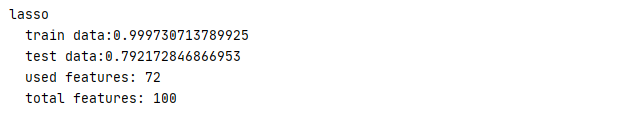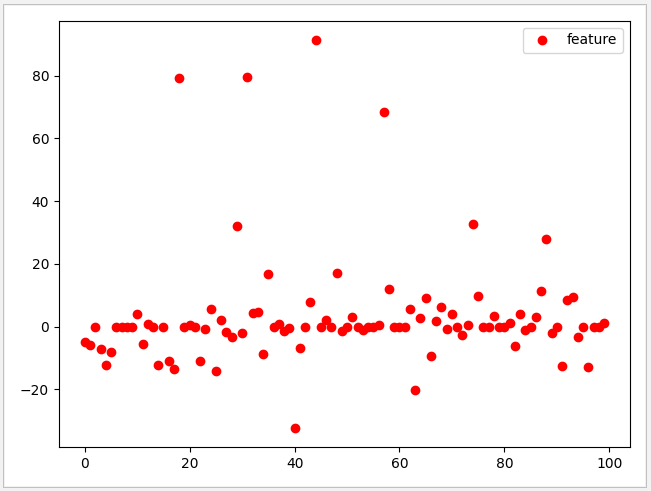试着把 alpha 调为2，此时有效特征下降到 45，而测试数据的分数达到 0.94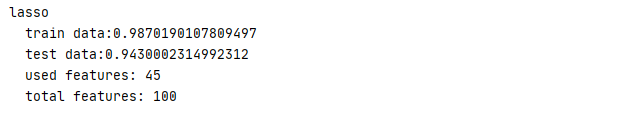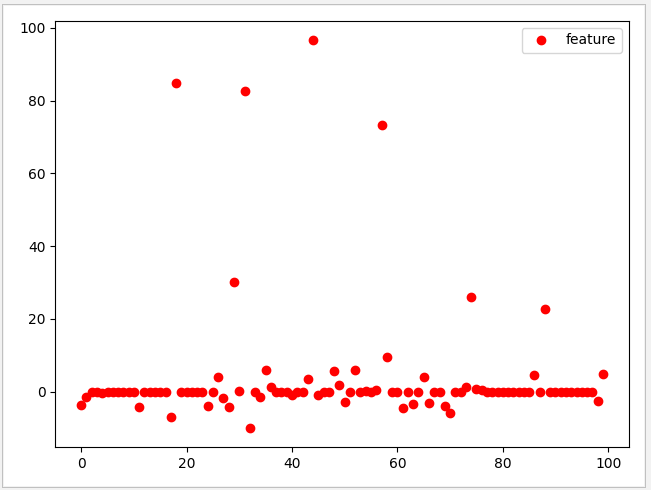4.4 浅谈正则化概念L2 正则化则是使用累加 W 平方值计算惩罚项，使用 L2 时 W(i) 的权重都不会为0，而是对每个元素进行不同比例的放缩。

 1 def ridge_test():
2     #测试数据
3     line=np.linspace(-3,3,100)
4     X,y=datasets.make_regression(n_features=100,noise=40,random_state=1)
5     X_train,X_test,y_train,y_test=train_test_split(X,y)
6     #岭回归模型
7     ridge=Ridge()
8     ridge.fit(X_train,y_train)
9     #计算准确率
10     print('ridge\n  train data:{0}'.format(ridge.score(X_train,y_train)))
11     print('  test data:'.format(ridge.score(X_test,y_test)))
12     print('  used features: {0}'.format(sum(ridge.coef_!=0)))
13     print('  total features: {0}'.format(ridge.n_features_in_))
14     plt.plot(ridge.coef_,'^',color='g')
15
16
17 def lasso_test():
18     # 测试数据
19     X,y=datasets.make_regression(n_features=100,noise=40,random_state=1)
20     X_train,X_test,y_train,y_test=train_test_split(X,y)
21     # 把alpha设置为2
22     lasso=Lasso(alpha=2,max_iter=10000)
23     lasso.fit(X_train,y_train)
24     #输出正确率
25     print('lasso\n  train data:{0}'.format(lasso.score(X_train,y_train)))
26     print('  test data:{0}'.format(lasso.score(X_test,y_test)))
27     #斜率与截距
28     print('  used features: {0}'.format(sum(lasso.coef_!=0)))
29     print('  total features: {0}'.format(lasso.n_features_in_))
30     plt.plot(lasso.coef_,'o',color='red')
31     plt.legend(['feature'])
32
33 ridge_test()
34 lasso_test()
35 plt.show()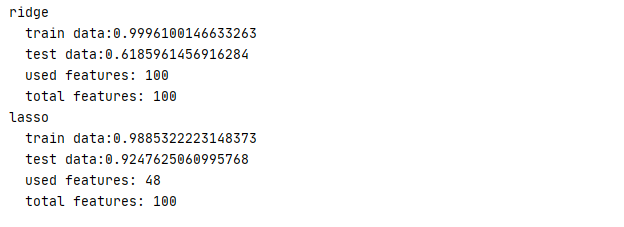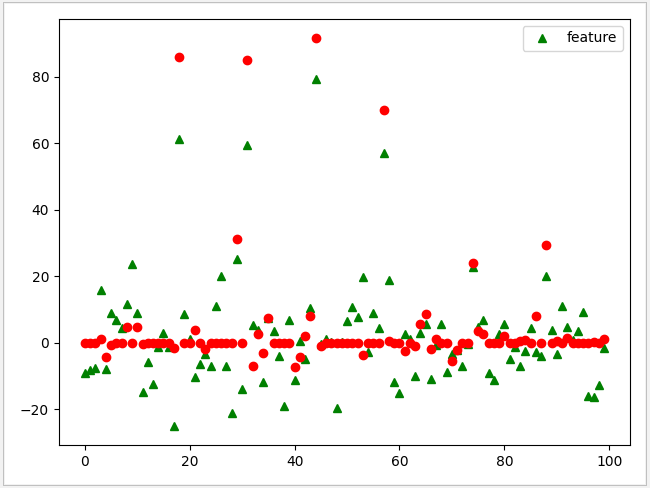4.5 SGDRegressor 模型

SGDRegressor 构造函数

1 class SGDRegressor(BaseSGDRegressor):
2     @_deprecate_positional_args
3     def __init__(self, loss="squared_loss", *, penalty="l2", alpha=0.0001,
4                  l1_ratio=0.15, fit_intercept=True, max_iter=1000, tol=1e-3,
5                  shuffle=True, verbose=0, epsilon=DEFAULT_EPSILON,
6                  random_state=None, learning_rate="invscaling", eta0=0.01,
7                  power_t=0.25, early_stopping=False, validation_fraction=0.1,
8                  n_iter_no_change=5, warm_start=False, average=False):
9      ......
• loss：默认为“squared_loss”, 选择要使用的损失函数。可用的回归损失函数有：'squared_loss'、'huber'、epsilon_unsensitive'或'squared_epsilon_unsensitive'。
• penalty：默认为 L2，用于指定惩罚项中使用的规范，可选参数为 L1 、 L2、elasticnet
• alpha：默认值为 0.0001 乘以正则项的常数。值越大，正则化越强。当学习率设为“optimal”时，用于计算学习率。
• l1_ratio：默认值为 0.15 弹性净混合参数，0 <= l1_ratio <= 1. l1_ratio=0对应于L2惩罚，l1_ratio=1到 l1。仅当 penalty 为 elasticnet 时使用。
• fit_intercept：bool类型，默认为True，表示是否计算截距 ( 即 y=wx+k 中的 k )。
• max_iter：默认值为 1000，部分求解器需要通过迭代实现，这个参数指定了模型优化的最大迭代次数。
• tol：默认值为1e-3,默认为小数点后 3 位，代表求解方法精度
• shuffle：默认值为 True ，是否在每个epoch之后对训练数据进行洗牌。
• verbose：默认值为 0 详细程度。
• epsilon：当 loss 选择 “huber” 时，它决定了一个阈值，在这个阈值下，预测值将被忽略。若选择 “epsilon-insensitive” 表示若当前预测和正确标签之间的差异小于此阈值，将被忽略。
• random_state：默认值为None 随机数种子，推荐设置一个任意整数，同一个随机值，模型可以复现。

1）‘constant’: eta = eta0；

2）‘optimal: eta = 1.0 / (alpha * (t + t0)) ;

3）‘invscaling’: eta = eta0 / pow(t, power_t);

• eta0：默认值为0.01，初始学习速率。
• power_t：默认值为0.25 反向缩放学习速率的指数
• early_stopping：默认值为 False 验证分数没有提高时，是否使用提前停止终止培训。如果设置为True，它将自动将训练数据的分层部分作为验证，并且当分数方法返回的验证分数对 n_iter_no_change 连续时间段没有至少提高tol时终止训练。
• validation_fraction：默认值为 0.1 作为早期停机验证设置的培训数据的比例。必须介于0和1之间。仅在“早停”为真时使用。
• n_iter_no_change：默认值为 5 在提前停止之前没有改进的迭代次数。
• warm_start：bool, 默认值为 False 当设置为True时，将上一个调用的解决方案重用为fit作为初始化，否则，只需删除以前的解决方案。
• average：默认值为 False 当设置为True时，计算所有更新的 averaged SGD权重，并将结果存储在coef_ 属性中。如果设置为大于1的整数，则当看到的样本总数达到平均值时，将开始平均。所以average=10将在看到10个样本后开始平均。

SGDRegressor 模型与 Ridge 类似，默认使用 L2 正则化，所有特征权重都不为 0 而是对每个元素进行不同比例的放缩。
max_iter  默认值为1000， 它标志着运行迭代的最大次数，有需要时可以增加 max_iter 提高准确率。

 1 def sgd_regressor_test():
2     # 测试数据
3     X,y=dataset.make_regression(n_samples=100,n_features=2)
4     X_train, X_test, y_train, y_test = train_test_split(X, y)
5     # SGD 模型
6     sgd = SGDRegressor(learning_rate='constant',eta0=0.001,average=True)
7     sgd.fit(X_train,y_train)
8     # 准确率
9     print('SGD:\n  train data:{0}\n  test data:{1}'
10         .format(sgd.score(X_train,y_train),sgd.score(X_test,y_test)))
11     ax=plt.axes(projection='3d')
12     print('  coef:{0}   intercept:{1}'.format(sgd.coef_,sgd.intercept_))
13     # 生成3维图
14     ax.scatter3D(X[:,0],X[:,1],y,color='red')
15     # 生成格网矩阵
16     x0, x1 = np.meshgrid(X[:,0], X[:,1])
17     z = sgd.coef_ * x0 + sgd.coef_ * x1+sgd.intercept_
18     # 绘制3d
19     ax.plot_surface(x0, x1, z,color='white',alpha=0.01)
20     plt.show()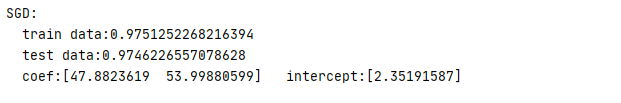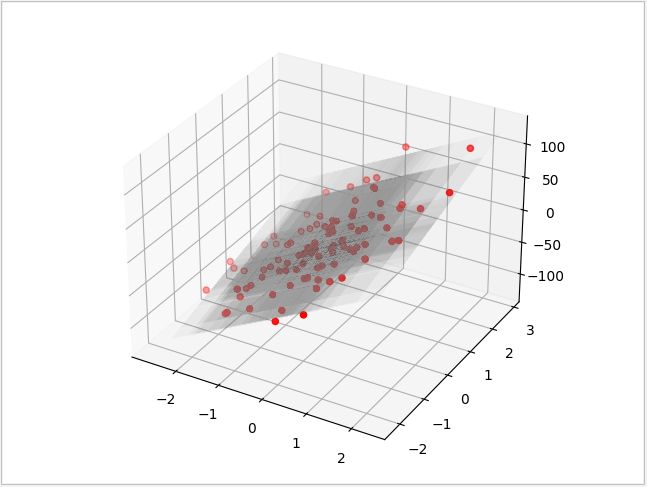1 def linear_regression_test():
2     # 测试数据
3     X, y = dataset.make_regression(n_samples=100000,n_features=100, random_state=2)
4     X_train, X_test, y_train, y_test = train_test_split(X, y)
5     # 线性回归模型训练
6     linear = LinearRegression()
7     linear.fit(X_train, y_train)
8     # 准确率
9     print('Linear:\n  train data:{0}\n  test data:{1}'
10           .format(linear.score(X_train,y_train),linear.score(X_test,y_test)))
11
12 def sgd_regressor_test():
13     # 测试数据
14     X,y=dataset.make_regression(n_samples=100000,n_features=100,random_state=2)
15     X_train, X_test, y_train, y_test = train_test_split(X, y)
16     # SGD 模型
17     sgd = SGDRegressor(learning_rate='constant',eta0=0.01)
18     sgd.fit(X_train,y_train)
19     # 准确率
20     print('SGD:\n  train data:{0}\n  test data:{1}'
21         .format(sgd.score(X_train,y_train),sgd.score(X_test,y_test)))
22
23 print('  Utime:{0}'.format(timeit.timeit(stmt=linear_regression_test, number=1)))
24 print('  Utime:{0}'.format(timeit.timeit(stmt=sgd_regressor_test, number=1)))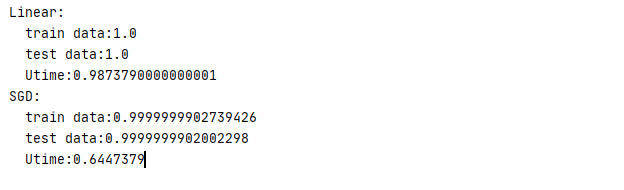4.6 LogisticRegression 模型

LogisticRegression 的构造函数

 1 class LogisticRegression(LinearClassifierMixin,
2                          SparseCoefMixin,
3                          BaseEstimator):
4 　　　　 @_deprecate_positional_args
5  　　　　def __init__(self, penalty='l2', *, dual=False, tol=1e-4, C=1.0,
6                  fit_intercept=True, intercept_scaling=1, class_weight=None,
7                  random_state=None, solver='lbfgs', max_iter=100,
8                  multi_class='auto', verbose=0, warm_start=False, n_jobs=None,
9                  l1_ratio=None):
10         ......

• penalty：默认为 L2，用于指定惩罚项中使用的规范，可选参数为 L1 和 L2。
• dual：默认为False，对偶或原始方法。对偶方法只用在求解线性多核(liblinear)的L2惩罚项上。当样本数量>样本特征的时候，dual通常设置为False。
• tol：默认为小数点后 4 位，代表求解方法精度。
• C：正则化系数 λ 的倒数，float类型，默认为1.0，越小的数值表示越强的正则化。
• fit_intercept：bool类型，默认为True，表示是否计算截距 ( 即 y=wx+k 中的 k )。
• intercept_scaling：float类型，默认为1，仅在 solver 为 ”liblinear”，且 fit_intercept设置为True时有用 。
• class_weight：用于标示分类模型中各种类型的权重，默认值为None，即不考虑权重。也可选择balanced 让类库自己计算类型权重，此时类库会根据训练样本量来计算权重，某种类型样本量越多，则权重越低，样本量越少，则权重越高。或者输入类型的权重比，例如 class_weight={0:0.9, 1:0.1}，此时类型0的权重为90%，而类型1的权重为10%。
• random_state：随机数种子，推荐设置一个任意整数，同一个随机值，模型可以复现。
• solver：求解优化算法，默认值 lbfgs，可以根据数据类型选择最合适的算法。可选的算法有：

1）liblinear：使用了开源的liblinear库实现，内部使用了坐标轴下降法来迭代优化损失函数。

2）lbfgs：利用损失函数二阶导数矩阵即海森矩阵来迭代优化损失函数。

3）newton-cg：利用损失函数二阶导数矩阵即海森矩阵来迭代优化损失函数。

4）sag：即随机平均梯度下降，是梯度下降法的变种，每次迭代仅仅用一部分的样本来计算梯度，适合数据量较大时使用。

5）saga：线性收敛的随机优化算法的的变重。

• max_iter：默认值为 100，部分求解器需要通过迭代实现，这个参数指定了模型优化的最大迭代次数。
• multi_class：分类方式选择参数，str类型，可选参数为ovr和multinomial，默认为ovr。如果是二元逻辑回归，ovr和multinomial并没有任何区别，区别主要在多元逻辑回归上。
• verbose：日志冗长度，int类型。默认为0。就是不输出训练过程，1的时候偶尔输出结果，大于1，对于每个子模型都输出。
• warm_start：默认值为 False，当设置为True时，重用之前调用的解决方案作为初始化，否则，只需要删除前面的解决方案。
• n_jobs：CPU 并行数，默认为None，代表1。若设置为 -1 的时候，则用所有 CPU 的内核运行程序。
• l1_ratios ： 默认为None，表示弹性网混合参数列表。

LogisticRegression 包含两个最常用的参数 penalty 正则化规则、C 正则化程度，由构造函数可以看到，一般情况下 LogisticRegression 使用的是 L2 正则规范。此时决定正则化规范强度的参数是由 C 值决定，C 越大，对应的正则化越弱，数据集的拟合度会更高，C 越小，则模型的权重系统 w 更趋向于 0。

 1 def logistic():
2     #生成数据集
3     X,y=datasets.make_forge()
4     X_train,X_test,y_train,y_test=train_test_split(X,y)
5     #对Logistic模型进行训练
6     logistic=LogisticRegression(C=1.0,random_state=1)
7     logistic.fit(X_train,y_train)
8     #输入正确率
9     print('logistic\n  train data:{0}'.format(logistic.score(X_train,y_train)))
10     print('  test data:{0}'.format(logistic.score(X_test,y_test)))
11     #输出模型点
12     plt.scatter(X[:,0], X[:,1],c=y,s=100)
13     plt.legend(['model','data'])
14     #输出模型决策边界
15     line = np.linspace(7, 13, 100)
16     y=(-logistic.coef_*line-logistic.intercept_)/logistic.coef_
17     plt.plot(line,y,'-')
18     plt.show()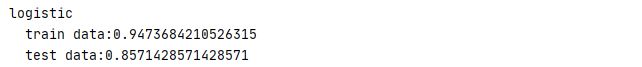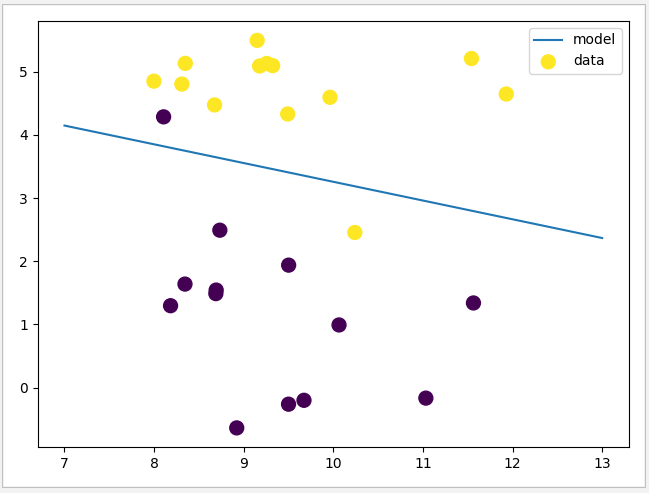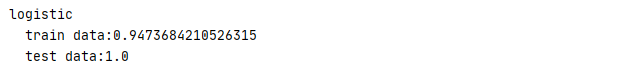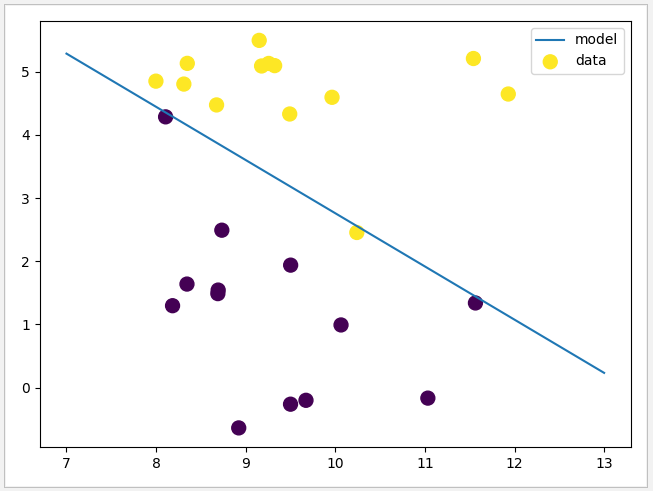相反，若将 C 调整为 0.01 时，权重系数 w 会越趋向于0，它会让公式适用于更多的数据点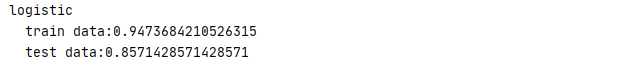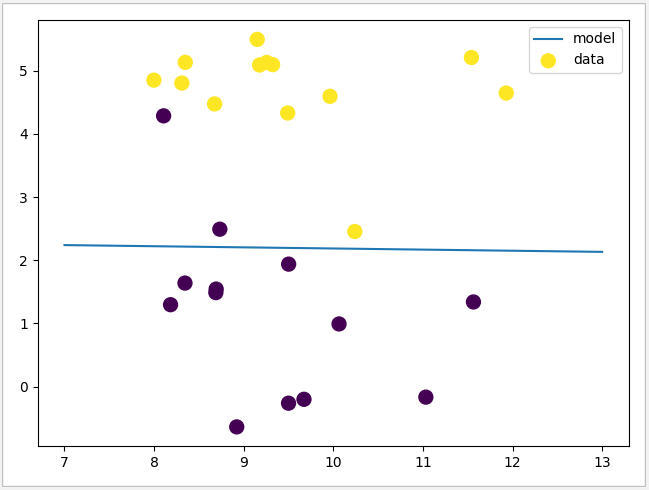LogisticRegression 除了可以通过 C 控制其正则化强度外，还可以通过 penalty 参数选择正则化规则，penalty 默认值为 L2，但是当数据集的特征较多时，可以尝试通过设置 penalty 让其使用 L1 正则化。但需要注意的是，当使用 L1 正则化时 solver 必须使用 liblinear 算法，否则系统会报错。

 1 def logistic(penalty,c):
2     #生成数据集
3     X,y=dataset.make_classification(n_samples=1000,n_features=100,random_state=1)
4     X_train,X_test,y_train,y_test=train_test_split(X,y)
5     #对Logistic模型进行训练
6     if(penalty=='l1'):
7         solver='liblinear'
8     else:
9         solver='lbfgs'
10     logistic=LogisticRegression(penalty=penalty,C=c,solver=solver,random_state=1)
11     logistic.fit(X_train,y_train)
12     #输入正确率
13     print('{0}\n  train data:{1}'.format(penalty,logistic.score(X_train,y_train)))
14     print('  test data:{0}'.format(logistic.score(X_test,y_test)))
15
16     #输出模型决策边界
17     print('  total features:{0}'.format(logistic.n_features_in_))
18     print('  used features:{0}'.format(sum(logistic.coef_!=0)))
19     plt.plot(logistic.coef_,'^')
20 logistic('l1',0.2)
21 logistic('l2',0.2)
22 plt.show()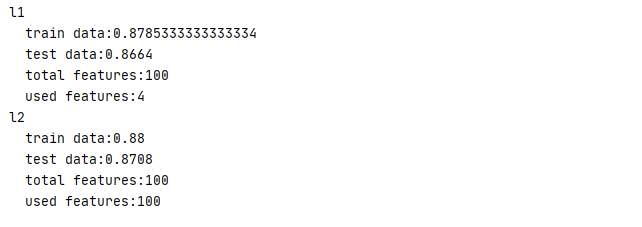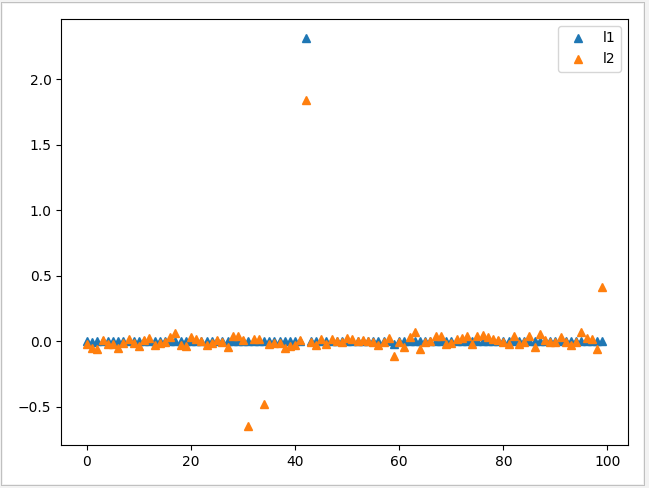1 logistic("l2", 0.01)
2 logistic('l2',100)
3 plt.legend(['c=0.01','c=100'])
4 plt.show()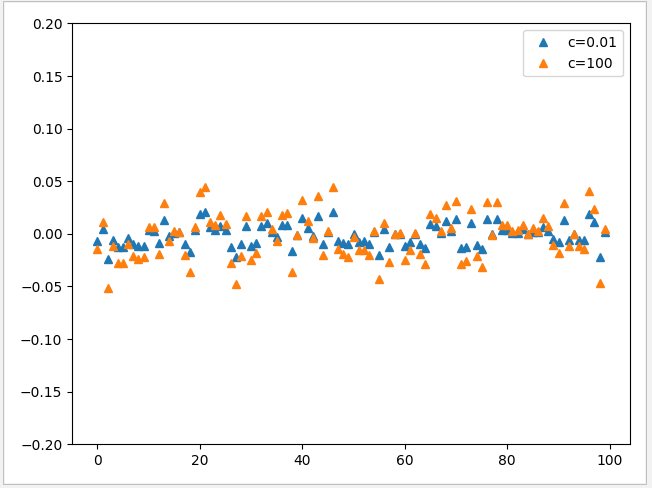可见 C 值越小，w 就会越趋向于0，C 值越大，w 就会越分散且受正则化的约束越小

4.7 LinearSVC 线性支持向量机

LinearSVC 的构造函数

1 class LinearSVC(LinearClassifierMixin,
2                 SparseCoefMixin,
3                 BaseEstimator):
4     @_deprecate_positional_args
5     def __init__(self, penalty='l2', loss='squared_hinge', *, dual=True,
6                  tol=1e-4, C=1.0, multi_class='ovr', fit_intercept=True,
7                  intercept_scaling=1, class_weight=None, verbose=0,
8                  random_state=None, max_iter=1000):
9         ......

• penalty：默认为 L2，用于指定惩罚项中使用的规范，可选参数为 L1 和 L2。当使用 L1 准则时，参数 loss 必须为 squared_hinge , dual 必须为 False。
• loss : 指定损失函数，默认值为 squared_hinge，可选择 ‘hinge’ 或 ‘squared_hinge’ 。
• dual：默认为False，对偶或原始方法。对偶方法只用在求解线性多核(liblinear)的L2惩罚项上。当样本数量>样本特征的时候，dual通常设置为False。
• tol：默认为小数点后 4 位，代表求解方法精度。
• C：正则化系数 λ 的倒数，float类型，默认为1.0，越小的数值表示越强的正则化。
• multi_class : 默认值为 ovr，可选择 ‘ovr’ 或 ‘crammer_singer’ ，用于确定多类策略。 “ovr” 训练n_classes one-vs-rest 分类器，而 “crammer_singer” 优化所有类的联合目标。  如果选择“crammer_singer”，则将忽略选项 loss，penalty 和 dual 参数。
• fit_intercept：bool类型，默认为True，表示是否计算截距 ( 即 y=wx+k 中的 k )。
• intercept_scaling：float类型，默认为1，仅在 fit_intercept设置为True时有用 。
• class_weight：用于标示分类模型中各种类型的权重，默认值为None，即不考虑权重。也可选择balanced 让类库自己计算类型权重，此时类库会根据训练样本量来计算权重，某种类型样本量越多，则权重越低，样本量越少，则权重越高。或者输入类型的权重比，例如 class_weight={0:0.9, 1:0.1}，此时类型0的权重为90%，而类型1的权重为10%。
• verbose：日志冗长度，int类型。默认为0。就是不输出训练过程，1的时候偶尔输出结果，大于1，对于每个子模型都输出
• random_state：随机数种子，推荐设置一个任意整数，同一个随机值，模型可以复现。
• max_iter：默认值为 10000，部分求解器需要通过迭代实现，这个参数指定了模型优化的最大迭代次数。

 1 def linearSVC():
2     #生成数据集
3     X,y=mglearn.datasets.make_blobs(n_samples=200,random_state=23,centers=3)
4     X_train,X_test,y_train,y_test=train_test_split(X,y)
5     #使用 LinearSVC 模型，使用 L1 准则
6     linearSVC=LinearSVC(penalty='l1',loss='squared_hinge', dual=False)
7     linearSVC.fit(X_train,y_train)
8     #输出准确率
9     print('LinearSVC\n  train data:{0}'.format(linearSVC.score(X_train,y_train)))
10     print('  test data:{0}'.format(linearSVC.score(X_test,y_test)))
11     #划出图形分隔线
12     plt.scatter(X[:,0], X[:,1],c=y,s=100,cmap='autumn',marker='*')
13     n=np.linspace(-8,8,100)
14     value0=(-n*linearSVC.coef_ - linearSVC.intercept_) / linearSVC.coef_
15     value1=(-n*linearSVC.coef_ - linearSVC.intercept_) / linearSVC.coef_
16     value2=(-n*linearSVC.coef_ - linearSVC.intercept_) / linearSVC.coef_
17     plt.plot(n.reshape(-1, 1), value0,'-')
18     plt.plot(n.reshape(-1, 1), value1,'--')
19     plt.plot(n.reshape(-1, 1), value2,'+')
20     plt.legend(['class0','class1','class2'])
21     plt.show()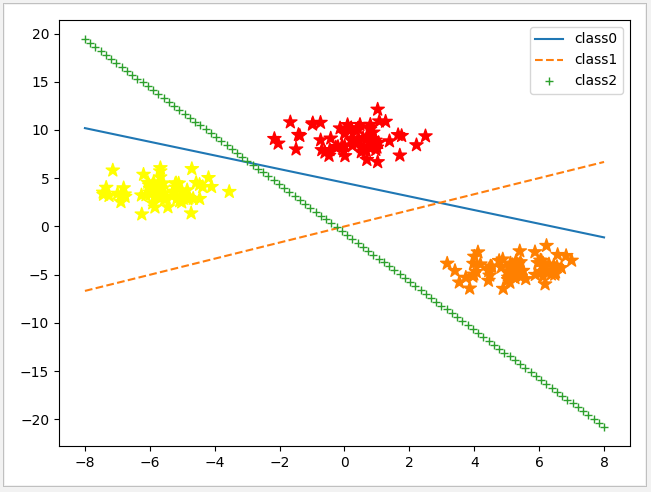4.8 SGDClassifier 分类模型

SGDClassifier 与 SGDRegressor 类似，都是使用梯度下降法进行分类计算，由于SGD是以一次一个的方式独立处理训练实例，所以它能够有效处理大型的数据集。SDGClassifier 默认也是使用 L2 准则，注意与SGDRegressor不同的是它的学习率 learning_rate 默认使用 optimal，此时 eta0 无效，若要使用 eta 0 需要提前修改 learning_rate 参数。

 1 class SGDClassifier(BaseSGDClassifier):
2     @_deprecate_positional_args
3     def __init__(self, loss="hinge", *, penalty='l2', alpha=0.0001,
4                  l1_ratio=0.15,
5                  fit_intercept=True, max_iter=1000, tol=1e-3, shuffle=True,
6                  verbose=0, epsilon=DEFAULT_EPSILON, n_jobs=None,
7                  random_state=None, learning_rate="optimal", eta0=0.0,
8                  power_t=0.5, early_stopping=False, validation_fraction=0.1,
9                  n_iter_no_change=5, class_weight=None, warm_start=False,
10                  average=False):
11     ......
• loss：默认为“hinge”, 选择要使用的损失函数。可用的损失函数有：'hinge', 'log', 'modified_huber','squared_hinge', 'perceptron'。log 损失使逻辑回归成为概率分类器。 'modified_huber'是另一个平滑的损失，它使异常值和概率估计具有一定的容忍度。“ squared_hinge”与hinge类似，但会受到二次惩罚。“perceptron”是感知器算法使用的线性损失。
• penalty：默认为 L2，用于指定惩罚项中使用的规范，可选参数为 L1 、 L2、elasticnet
• alpha：默认值为 0.0001 乘以正则项的常数。值越大，正则化越强。当学习率设为“optimal”时，用于计算学习率。
• l1_ratio：默认值为 0.15 弹性净混合参数，0 <= l1_ratio <= 1. l1_ratio=0对应于L2惩罚，l1_ratio=1到 l1。仅当 penalty 为 elasticnet 时使用。
• fit_intercept：bool类型，默认为True，表示是否计算截距 ( 即 y=wx+k 中的 k )。
• max_iter：默认值为 1000，部分求解器需要通过迭代实现，这个参数指定了模型优化的最大迭代次数。
• tol：默认值为1e-3,默认为小数点后 3 位，代表求解方法精度
• shuffle：默认值为 True ，是否在每个epoch之后对训练数据进行洗牌。
• verbose：默认值为 0 详细程度。
• epsilon：默认值为0.1  loss 选择 “huber” 时，它决定了一个阈值，在这个阈值下，预测值将被忽略。若选择 “epsilon-insensitive” 表示若当前预测和正确标签之间的差异小于此阈值，将被忽略。
• n_job：CPU 并行数，默认为None，代表1。若设置为 -1 的时候，则用所有 CPU 的内核运行程序。
• random_state：默认值为None 随机数种子，推荐设置一个任意整数，同一个随机值，模型可以复现。

1）‘constant’: eta = eta0；

2）‘optimal: eta = 1.0 / (alpha * (t + t0)) ;

3）‘invscaling’: eta = eta0 / pow(t, power_t);

• eta0：默认值为0.0，初始学习速率。当 learning_rate 为 optimal 时，此值无效。
• power_t：默认值为0.5 反向缩放学习速率的指数
• early_stopping：默认值为 False 验证分数没有提高时，是否使用提前停止终止培训。如果设置为True，它将自动将训练数据的分层部分作为验证，并且当分数方法返回的验证分数对 n_iter_no_change 连续时间段没有至少提高tol时终止训练。
• validation_fraction：默认值为 0.1 作为早期停机验证设置的培训数据的比例。必须介于0和1之间。仅在“早停”为真时使用。
• n_iter_no_change：默认值为 5 在提前停止之前没有改进的迭代次数。
• class_weight:  类别关联的权重，使用字典格式，默认值 {class_label: None} 也可选择balanced 让类库自己计算类型权重，此时类库会根据训练样本量来计算权重，某种类型样本量越多，则权重越低，样本量越少，则权重越高。或者输入类型的权重比，例如 class_weight={0:0.9, 1:0.1}，此时类型0的权重为90%，而类型1的权重为10%。
• warm_start：bool, 默认值为 False 当设置为True时，将上一个调用的解决方案重用为fit作为初始化，否则，只需删除以前的解决方案。
• average：默认值为 False 当设置为True时，计算所有更新的 averaged SGD权重，并将结果存储在coef_ 属性中。如果设置为大于1的整数，则当看到的样本总数达到平均值时，将开始平均。所以average=10将在看到10个样本后开始平均。

 1 def sgd_classifier_test():
2     # 输入入数据
4     # 把28*28图像数据进行转换
5     X_train=X_train.reshape(-1,784)
6     X_test=X_test.reshape(-1,784)
7     #使用SGDClassfier模式，使用多核计算,学习率为0.01
8     sgd_classifier=SGDClassifier(learning_rate='constant',eta0=0.01,n_jobs=-1)
9     sgd_classifier.fit(X_train,y_train)
10     #查看准确率
11     print('SGDClassfier\n  train data:{0}\n  test data:{1}'.format(
12         sgd_classifier.score(X_train,y_train)
13         ,sgd_classifier.score(X_test,y_test)))
14     #查看测试数量第256幅图
15     data=X_test.reshape(28,28)
16     plt.imshow(data,cmap='binary')
17     plt.show()
18     print('  test number is:{0}'.format(y_test))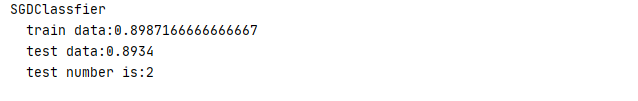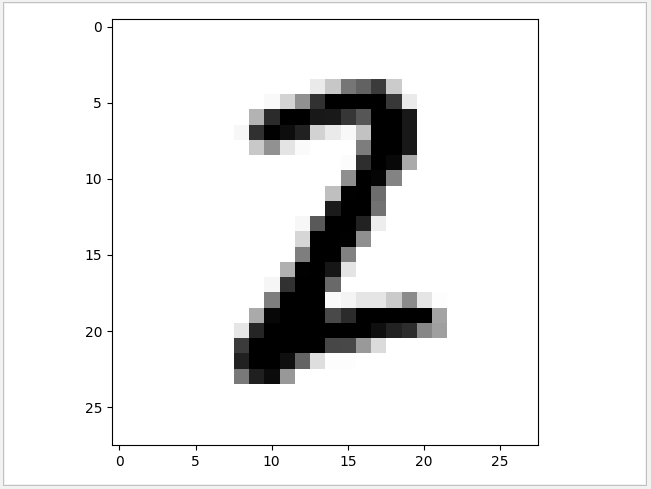4.9 多项式转换器与管道

4.9.1 PolynomialFeatures 转换器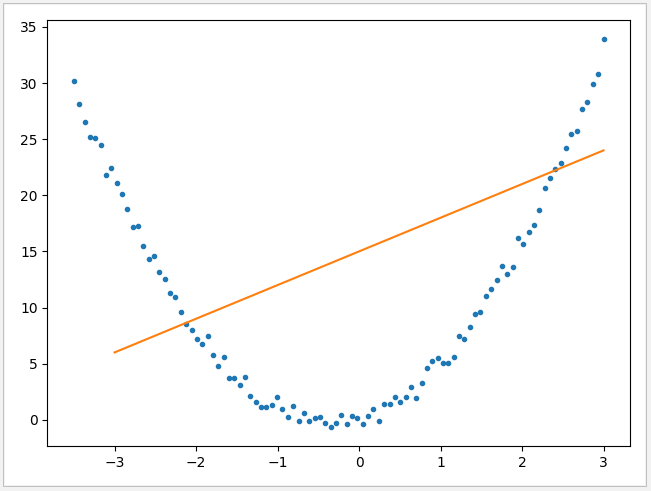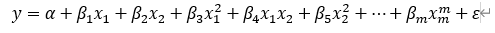1 class PolynomialFeatures():
2     @_deprecate_positional_args
3     def __init__(self, degree=2, *, interaction_only=False, include_bias=True,
4                  order='C'):
5         self.degree = degree
6         self.interaction_only = interaction_only
7         self.include_bias = include_bias
8         self.order = order
9         ......
• degree：默认值为2，控制多项式的次数；
• interaction_only：默认为 False，如果指定为 True，那么就不会有特征自己和自己结合的项，组合的特征中没有 X12X1 * X23
• include_bias：默认为 True 。如果为 True 的话，那么结果中就会有 0 次幂项，即全为 1 这一列。
• order:  默认为"C" ，可选择 "F" 。“C” 表示是在密集情况（dense case）下的输出array的顺序，“F” 可以加快操作但可能使得subsequent estimators变慢。

 1 # 测试数据，根据 y=3*x*x+2*x+1 生成
2 def getData():
3     x=np.linspace(-3.5,3,100)
4     y=3*x*x+2*x+1
5     d=np.random.random(100)*2
6     y=y-d
7     plt.plot(x,y,'.')
8     return [x,y]
9
10 def polynomial_test():
11     # 获取测试数据
12     data=getData()
13     X=data.reshape(-1,1)
14     y=data
15     # 生成多项式回归模型
16     polynomial=PolynomialFeatures(degree=2)
17     X_poly=polynomial.fit_transform(X)
18     # 把运算过的数据放到 LinearRegression 进行运算
19     linearRegression=LinearRegression()
20
21     linearRegression.fit(X_poly,y)
22     # 打印数据
23     print('PolynomialFeature:\n  coef:{0}\n  intercept:{1}\n  score:{2}'
24           .format(linearRegression.coef_,linearRegression.intercept_,
25                   linearRegression.score(X_poly,y)))
26     # 根据斜率和截距画出图
27     x=np.linspace(-3.5,3,100)
28     y=linearRegression.coef_*x*x+linearRegression.coef_*x+linearRegression.intercept_
29     plt.plot(x,y)
30     plt.legend(['data','model'])
31     plt.show()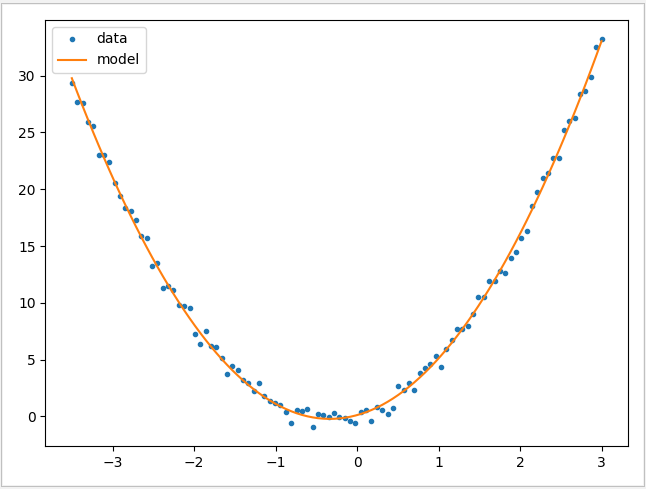可见使用 PolynomialFeatures 多项式转换器可以向数据中加入非线性特征，让线性模型变得更加强大。

4.9.2 Pipeline 管道

 1 # 测试数据根据 y=3*x*x+2*x+1 生成
2 def getData():
3     x=np.linspace(-3.5,3,100)
4     y=3*x*x+2*x+1
5     d=np.random.random(100)*2
6     y=y-d
7     plt.plot(x,y,'.')
8     return [x,y]
9
10 def polynomial_test():
11     # 获取测试数据
12     data=getData()
13     X=data.reshape(-1,1)
14     y=data
15     # 生成管道先执行 PolynomialFeatures 再执行 LinearRegression
16     pipe=make_pipeline(PolynomialFeatures(degree=2),LinearRegression())
17     # 训练数据
18     pipe.fit(X,y)
19     # 获取执行对象
20     linearRegression=pipe.steps
21     # 打印数据
22     print('PolynomialFeature:\n  coef:{0}\n  intercept:{1}\n  score:{2}'
23           .format(linearRegression.coef_,linearRegression.intercept_,
24                   pipe.score(X,y)))
25     # 根据斜率和截距画出图
26     x=np.linspace(-3.5,3,100)
27     y=linearRegression.coef_*x*x+linearRegression.coef_*x+linearRegression.intercept_
28     plt.plot(x,y)
29     plt.legend(['data','model'])
30     plt.show()

AI人功智能相关文章

Python 机器学习实战 —— 监督学习（上）

Python 机器学习实战 —— 监督学习（下）

Python 机器学习实战 —— 无监督学习（上）

Python 机器学习实战 —— 无监督学习（下）

Tensorflow 2.0 深度学习实战——介绍损失函数、优化器、激活函数、多层感知机的实现原理

https://www.cnblogs.com/leslies2/p/14832685.html

posted on 2021-06-17 10:48  风尘浪子  阅读(644)  评论(2编辑  收藏  举报